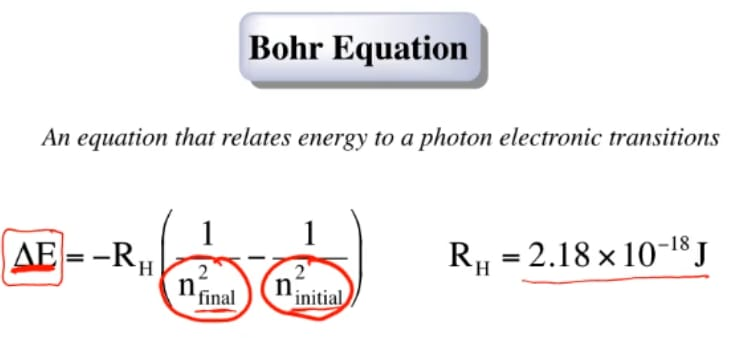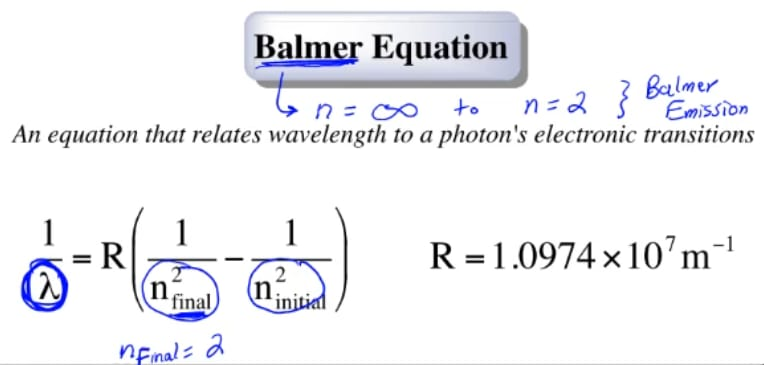Clutch Prep is now a part of Pearson
Ch.7 - Quantum MechanicsWorksheetSee all chapters

# Bohr Equation

See all sections
Sections
Wavelength and Frequency
Speed of Light
The Energy of Light
Electromagnetic Spectrum
Photoelectric Effect
De Broglie Wavelength
Heisenberg Uncertainty Principle
Bohr Model
Emission Spectrum
Bohr Equation
Introduction to Quantum Mechanics
Quantum Numbers: Principal Quantum Number
Quantum Numbers: Angular Momentum Quantum Number
Quantum Numbers: Magnetic Quantum Number
Quantum Numbers: Spin Quantum Number
Quantum Numbers: Number of Electrons
Quantum Numbers: NodesJules Bruno

# Bohr and Balmer equations

Both Bohr’s equation and Balmer’s equation try their best to describe and characterize changes in either energy or wavelength when a photon or an electron goes from one shell to another shell.

## Bohr equation

In the Bohr equation, we’re going to say this is an equation that relates energy to a photon electronic transitions. We’re going to say here we’re talking about an electron or photon going from one shell to another shell and how that affects the energy (either absorbed or released).

The equation is delta E = RH (1/n2final - 1/n2 initial).

Here, our RH value is 2.18 x 10-18 Joules. We’d use this version of the Bohr equation when they’re giving us or discussing two shells like you’re going from shell 3 to shell 1 and they reference energy. Again, if they’re talking about two shells, and they’re referencing energy, we use Bohr’s equation.## Balmer rydberg equation

In Balmer’s equation, this equation relates wavelength to a photon’s electronic transitions. In the above example, it tries to relate changes in your shell number to the wavelength. Here, R now becomes 1.0974 x 107 m-1. Realize here that when we use the term Balmer’s equation, Balmer in terms of emissions which means we’re going from a  higher shell number to a lower shell number, it usually means we’re starting off at a higher shell number and we’re going down to the second shell. That represents a Balmer emission. That deals with the origin of this equation.

When they were talking about Balmer’s equation, the final n final would’ve been 2. It doesn't necessarily always have to equal 2 now because they could give you a different value for the final shell number. Just realize that in the origins of this equation are

n final = 2. This is because a Balmer emission is going from a higher shell number down to shell number 2, making shell number 2 our final shell.## Summary

Remember that with Bohr’s equation and Balmer’s equation, they try to understand what’s happening to a photon as it goes from one shell to another. With Bohr’s equation, we’re relating shell numbers to energy. With the Balmer equation, we’re relating shell numbers to wavelength.Jules Bruno

Jules felt a void in his life after his English degree from Duke, so he started tutoring in 2007 and got a B.S. in Chemistry from FIU. He’s exceptionally skilled at making concepts dead simple and helping students in covalent bonds of knowledge.